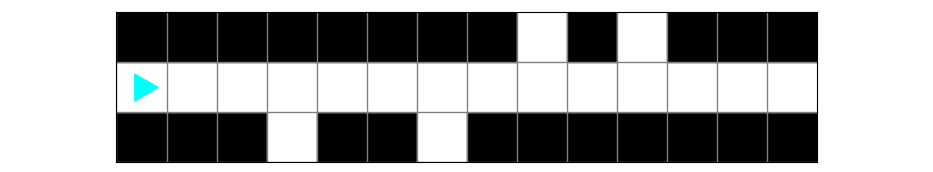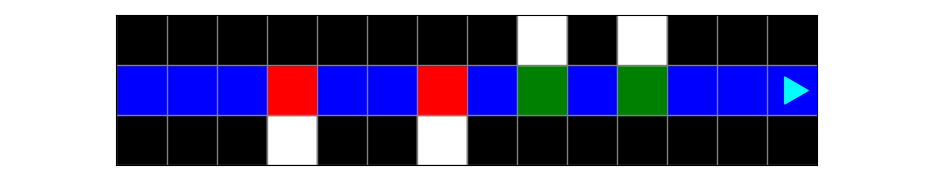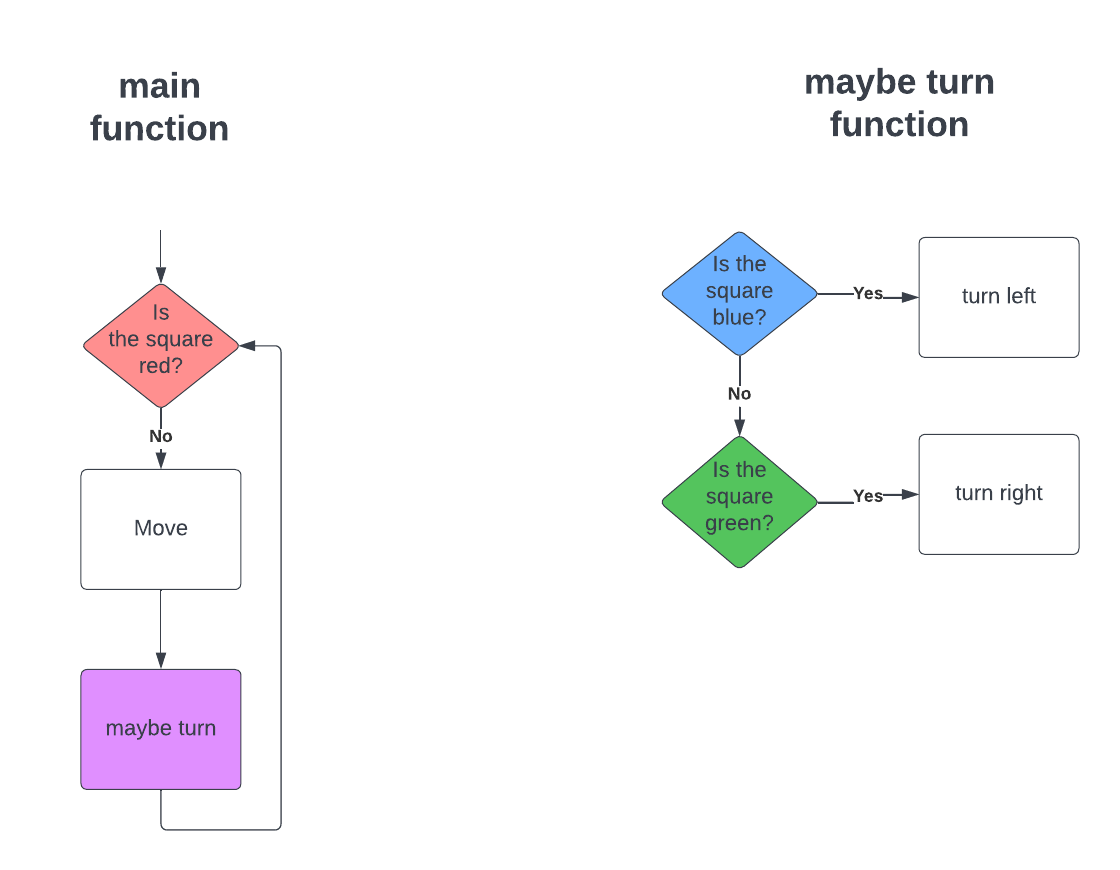Computer Science

# Practice with if statements

These problems will give you a chance to practice using `if` statements. Use the `event stream` pattern, and think carefully about when you need to use `elif` and `else`.

Download the zip file above and put it in your `bit` folder.

## Holes

For this problem, Bit starts in this world:Bit is a pipe, and the pipe has holes on each side. Bit’s job is to mark where the holes are so someone else can fix them. Bit goes down the center and follows these rules:

• mark holes on the right by painting the current square red
• mark holes on the left by painting the current square green
• otherwise paint squares blue

The ending world should look like this:You will find starter code in `holes.py`:

from byubit import Bit

``````@Bit.worlds('holes')
def run(bit):
# Write code here
pass

if __name__ == '__main__':
run(Bit.new_bit)``````

You should use the `event stream` pattern to solve this problem.

### Flow chart

A good way to think about problems that use `if` statements is to use a flow chart:In a flow chart, the rectangles represent a `process` — something you do — and the triangles represent a `decision` — a condition for a while loop or if statement.

The purple square represents a separate function we have to write. We can call this a `helper` function, because it helps the main function do its job.

In this flow chart, we have separated the main function into the left side and a helper function on the right side. This helps make the event stream pattern clear. The main function moves forward, and the helper function handles the event stream.

### Writing code

How would you turn this flow chart into code?One thing to spot from this flow chart is that we should have a while loop for the decision labeled `is the front clear?` because we loop back to check this repeatedly. We should have an `if-elif-else` statement for the other three decisions.

Here is one way to do this:

``````# Solution
from byubit import Bit

def change_square(bit):
if bit.right_clear():
bit.paint('red')
elif bit.left_clear():
bit.paint('green')
else:
bit.paint('blue')

@Bit.worlds('holes')
def run(bit):
bit.paint('blue')
while bit.front_clear():
bit.move()
change_square(bit)

if __name__ == '__main__':
run(Bit.new_bit)
``````

Run this code and use the buttons to step through it carefully to be sure you understand how `if` statements work.

## Fly

In this problem, Bit starts in this world:Bit is flying around and follows these rules:

• when Bit finds a blue square, it turns left.

• when Bit finds a green square, it turns right.

• when Bit finds a red square, it stops.### Planning

How would you turn these rules into a flow chart?Here is one way to do this:The import thing to recognize here is that the main function checks whether the current square is red. This is because of the final rule listed above — when Bit finds a red square, it stops. Instead of moving while the front is clear, we move while the current square is not red.

We are again using the `event stream` pattern, with a main function that moves forward and a helper function that turns as needed.

### Coding

Can you turn this into code?Notice that the main function moves while the current square is not red. This is a signal that you need to use `while not bit.is_red()`. Here is the code:

``````# Solution
from byubit import Bit

def maybe_turn(bit):
if bit.is_blue():
bit.left()
elif bit.is_green():
bit.right()

@Bit.worlds('fly')
def run(bit):
while not bit.is_red():
bit.move()
maybe_turn(bit)

if __name__ == '__main__':
run(Bit.new_bit)``````

The `if` statement uses `elif` but does not need an `else`.

We could have also written the `maybe_turn()` function as:

``````def maybe_turn(bit):
if bit.is_blue():
bit.left()
if bit.is_green():
bit.right()``````

This works because Bit can never be on a square that is both blue and green at the same time.

We could also have reversed the `bit.move()` and the `maybe_turn()`:

``````def run(bit):
while not bit.is_red():
maybe_turn(bit)
bit.move()``````

The solution works whether Bit moves and then turns, or if Bit turns and then moves. This works because Bit does not start on a square that is blue or green. If it did, then you would want to check for a turn before moving.

Run the code above and use the buttons to be sure you understand how and why it works.

### Possible bugs

Try adding these bugs to the code above to see what happens:

1. In the main function, go forward while the front is clear.

2. In the `maybe_turn()` function, turn right on blue and left on green.

3. In the `maybe_turn()` function, use `else` instead of `elif`.

In each case, step through the code with `First` and `Next` to observe what happens.